## ML Aggarwal Class 10 Solutions for ICSE Maths Chapter 9 Arithmetic and Geometric Progressions Ex 9.4

These Solutions are part of ML Aggarwal Class 10 Solutions for ICSE Maths. Here we have given ML Aggarwal Class 10 Solutions for ICSE Maths Chapter 9 Arithmetic and Geometric Progressions Ex 9.4

More Exercises

Question 1.
Can 0 be a term of a geometric progression?
Solution:
No, 0 is not a term of geometric progression.

Question 2.
(i) Find the next term of the list of numbers $$\frac { 1 }{ 6 } ,\frac { 1 }{ 3 } ,\frac { 2 }{ 3 } ,…$$
(ii) Find the next term of the list of numbers $$\frac { 3 }{ 16 } ,-\frac { 3 }{ 8 } ,\frac { 3 }{ 4 } ,-\frac { 3 }{ 2 } ,…$$
(iii) Find the 15th term of the series $$\sqrt { 3 } +\frac { 1 }{ \sqrt { 3 } } +\frac { 1 }{ 3\sqrt { 3 } } +…$$
(iv) Find the nth term of the list of numbers $$\frac { 1 }{ \sqrt { 2 } } ,-2,4\sqrt { 2 } ,-16,…$$
(v) Find the 10th and nth terms of the list of numbers 5, 25, 125, …
(vi) Find the 6th and the nth terms of the list of numbers $$\frac { 3 }{ 2 } ,\frac { 3 }{ 4 } ,\frac { 3 }{ 8 } ,…$$
(vii) Find the 6th term from the end of the list of numbers 3, – 6, 12, – 24, …, 12288.
Solution:
(i) Given
⇒ $$\frac { 1 }{ 6 } ,\frac { 1 }{ 3 } ,\frac { 2 }{ 3 } ,…$$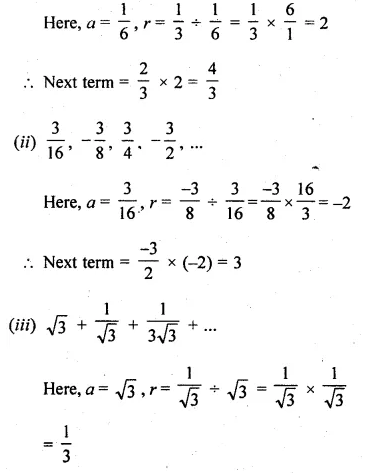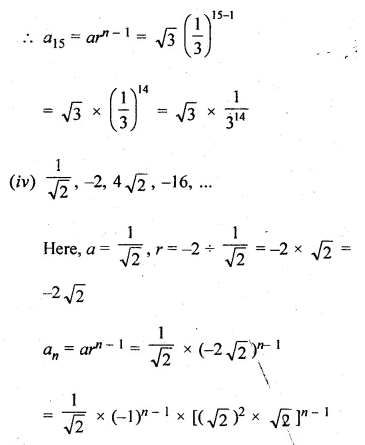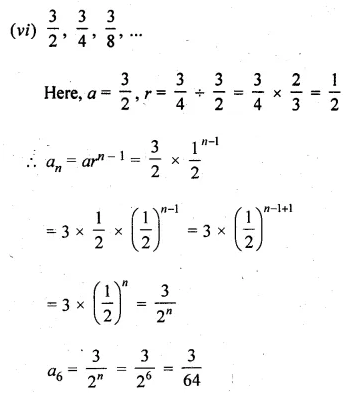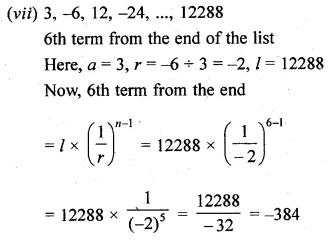Question 3.
Which term of the G.P.
(i) 2, 2√2, 4, … is 128?
(ii) $$1,\frac { 1 }{ 3 } ,\frac { 1 }{ 9 } ,…is\quad \frac { 1 }{ 243 } ?$$
(iii) $$\frac { 1 }{ 3 } ,\frac { 1 }{ 9 } ,\frac { 1 }{ 27 } ,…is\quad \frac { 1 }{ 19683 } ?$$
Solution:
Given
(i) 2, 2√2, 4, … is 128?
Here a = 2, $$r=\frac { 2\sqrt { 2 } }{ 2 } =\sqrt { 2 }$$, l = 128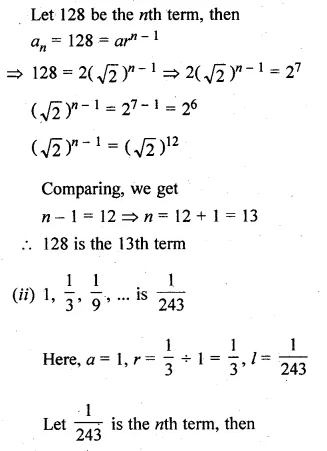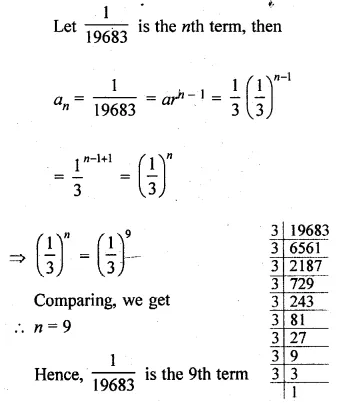Question 4.
Which term of the G.P. 3, – 3√3, 9, – 9√3, … is 729 ?
Solution:
G.P. 3, -3√3, 9, – 9√3, … is 729 ?
Here a = 3, $$r=\frac { -3\sqrt { 3 } }{ 3 } =\sqrt { -3 }$$, l = 729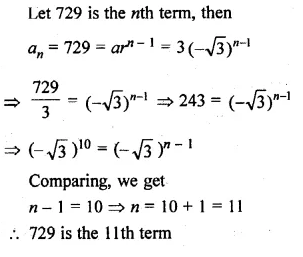Question 5.
Determine the 12th term of a G.P. whose 8th term is 192 and common ratio is 2.
Solution:
In a G.P.
a8 = 192 and r = 2
Let a be the first term and r be the common ratio then.∴ a12 = 3072

Question 6.
In a GP., the third term is 24 and 6th term is 192. Find the 10th term
Solution:
In a GP.
a3 = 24 and a6 = 192, a10 = ?
Let a be the first term and r be the common ratio, therefore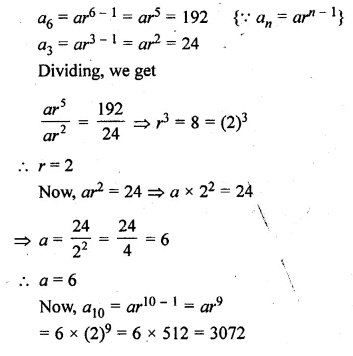Question 7.
Find the number of terms of a G.P. whose first term is $$\\ \frac { 3 }{ 4 }$$, common ratio is 2 and the last term is 384.
Solution:
First term of a G.P. (a) = $$\\ \frac { 3 }{ 4 }$$
and common ratio (r) = 2
Last term = 384
Let number of terms is n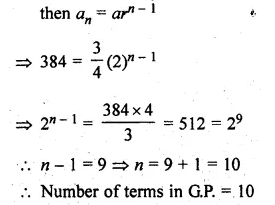Question 8.
Find the value of x such that
(i) $$-\frac { 2 }{ 7 } ,x,-\frac { 7 }{ 2 }$$ are three consecutive terms of a G.P.
(ii) x + 9, x – 6 and 4 are three consecutive terms of a G.P.
(iii) x, x + 3, x + 9 are first three terms of a G.P. Sol. Find the value of x
Solution:
Find the value of x
(i) $$-\frac { 2 }{ 7 } ,x,-\frac { 7 }{ 2 }$$ are three consecutive terms of a G.P.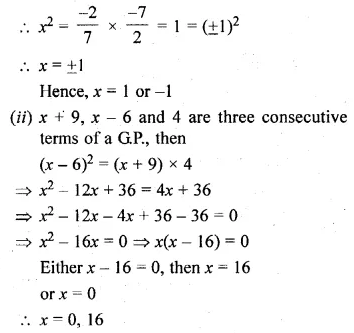Question 9.
If the fourth, seventh and tenth terms of a G.P. are x, y, z respectively, prove that x, y, z are in G.P.
Solution:
In a G.P.
a4 = x, a7 = y, a10 = z
To prove : x, y, z are in G.P.
Let a be the first term and r be the common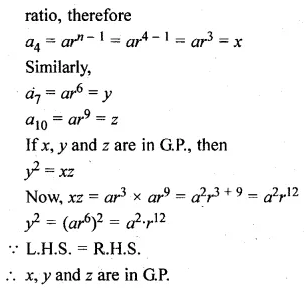Question 10.
The 5th, 8th and 11th terms of a G.P. are p, q and s respectively. Show that q² = ps.
Solution:
In a G.P.
a5 = p, a8 = q and a11 = s
To show that q² = px
Let a be the first term and r be the common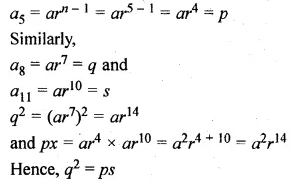Question 11.
If a, b, c are in G.P., then show that a², b², c² are also in G.P.
Solution:
a, b, c are in G.P.
Show that a², b², c² are also in G.P
∵ a, b, c are in G.P., then
b² = ac …(i)
a², b², c² will be in G.P.
if (b²)² = a² x c²
⇒ (ac)² = a²c² [From (i)]
⇒ a²c² = a²c² which is true.
Hence proved.

Question 12.
If a, b, c are in A.P., then show that 3a, 3b, 3c are in G.P.
Solution:
a, b and c are in A.P.
Then, 2b = a + c
Now, 3a, 3b, 3c will be in G.P.
if (3b)2 = 3a.3c
if 32b = 3a+c
Comparing, we get
if 2b = a + c
Which are in A.P. is given

Question 13.
If a, b, c are in A.P., then show that 10ax + 10, 10bx + 10, 10cx + 10, x ≠ 0, are in G.P.
Solution:
a, b, c are in A.P.
To show that are in G.P.10ax + 10, 10bx + 10, 10cx + 10, x ≠ 0
∵ a, b, c are in A.P.Question 14.
If a, a2+ 2 and a3 + 10 are in G.P., then find the values(s) of a.
Solution:
a, a2 + 2 and a3 + 10 are in G.P.
∵ (a2 + 2)2 = a(a3 + 10)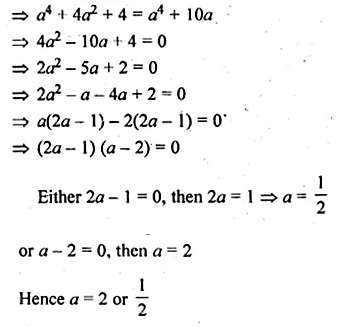Question 15.
If k, 2k + 2, 3k + 3, … are in G.P., then find the common ratio of the G.P.
Solution:
k, 2k + 2, 3k + 3, … are in G.P.
then, (2k + 2)2 = k(3k + 3)
⇒ 4k2 + 8k + 4 = 3k2 + 3k
⇒ 4k2 + 8k + 4 – 3k2 – 3k = 0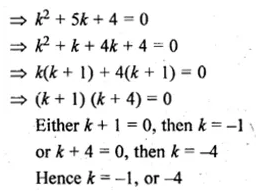Question 16.
The first and the second terms of a GP. are x-4 and xm . If its 8th term is x52, then find the value of m.
Solution:
In a G.P.,
First term (a1) = x-4 …(i)
Second term (a2) = xm
Eighth term (a8) = x52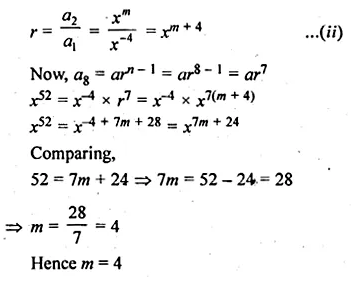Question 17.
Find the geometric progression whose 4th term is 54 and the 7th Term is 1458.
Solution:
In a G.P.,
4th term (a4) = 54Question 18.
The fourth term of a GP. is the square of its second term and the first term is – 3. Determine its seventh term.
Solution:
In a GP.
an is square of a2 i.e. an = (a2
a1 = – 3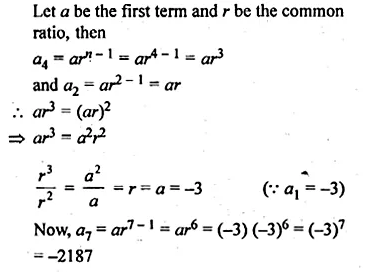Question 19.
The sum of first three terms of a G.P. is $$\\ \frac { 39 }{ 10 }$$ and their product is 1. Find the common ratio and the terms.
Solution:
Sum of first three terms of G.P. = $$\\ \frac { 39 }{ 10 }$$
and their product = 1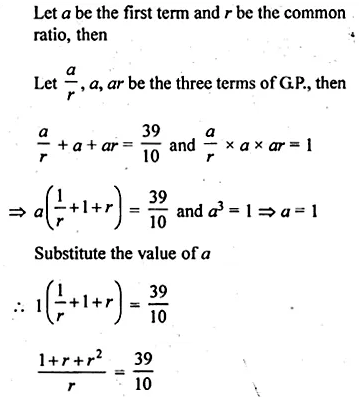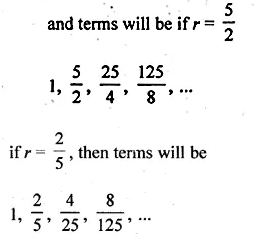Question 20.
Three numbers are in A.P. and their sum is 15. If 1, 4 and 19 are added to these numbers respectively, the resulting numbers are in G.P. Find the numbers.
Solution:
Given: Three numbers are in A.P. and their sum = 15
Let a – d, a, a + d be the three number in A.P.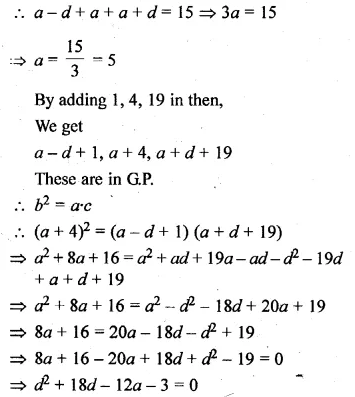Question 21.
Three numbers form an increasing G.P. If the middle term is doubled, then the new numbers are in A.P. Find the common ratio of the G.P.
Solution:
Three numbers form an increasing G.P.
Let $$\\ \frac { a }{ r }$$ ,a,ar be three numbers in G.P.
Double the middle term, we get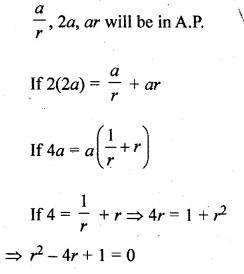Question 22.
Three numbers whose sum is 70 are in GP. If each of the extremes is multiplied by 4 and the mean by 5, the numbers will be in A.P. Find the numbers.
Solution:
Three numbers are in G.P.
Let numbers be
$$\\ \frac { r }{ a }$$, a, ar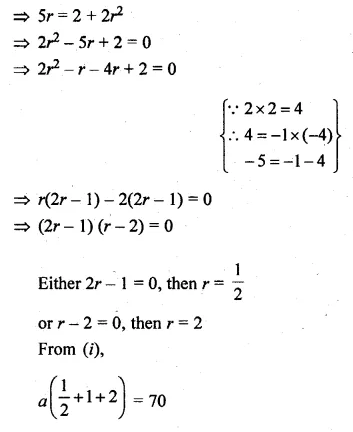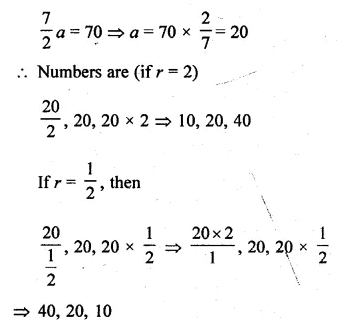Question 23.
There are four numbers such that first three of them form an A.P. and the last three form a GP. The sum of the first and third number is 2 and that of second and fourth is 26. What are these numbers?
Solution:
There are 4 numbers, such that
First 3 numbers are in A.P. and
last 3 numbers are in GP.
Sum of first and third numbers = 2
and sum of 2nd and 4th = 26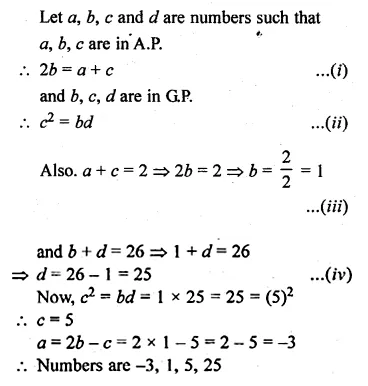Question 24.
(i) If a, b, c are in A.P. as well in G.P., prove that a = b = c.
(ii) If a, b, c are in A.P as well as in G.P., then find the value of ab-c + bc-a + ca-b
Solution:
(i) a, b, c are in A.P. as well as in GP.
To prove: a = b = c
a, b, c are in A.P.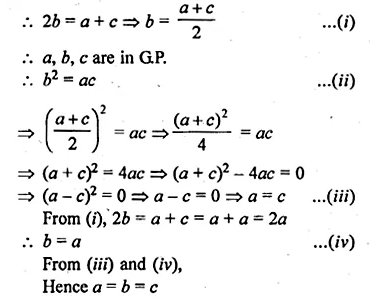Question 25.
The terms of a G.P. with first term a and common ratio r are squared. Prove that resulting numbers form a G.P. Find its first term, common ratio and the nth term.
Solution:
In a G.P.,
The first term = a
and common ratio = r
GP. is a, ar, ar²
Squaring we get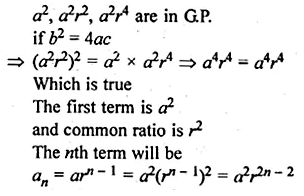Question 26.
Show that the products of the corresponding terms of two G.P.’s a, ar, ar², …, arn-1 and A, AR, AR2, …, ARn-1 form a G.P. and find the common ratio.
Solution:
It has to be proved that the sequence
aA, arAR, ar²AR2, …, arn-1ARn-1 and forms a G.P.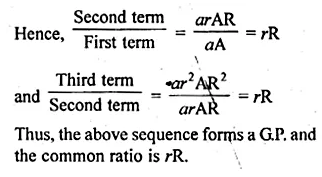Question 27.
(i) If a, b, c are in G.P. show that $$\frac { 1 }{ a } ,\frac { 1 }{ b } ,\frac { 1 }{ c }$$ are also in G.P.
(ii) If K is any positive real number and Ka, Kb Kc are three consecutive terms of a G.P., prove that a, b, c are three consecutive terms of an A.P.
(iii) If p, q, r are in A.P., show that pth, qth and rth terms of any G.P. are themselves in GP.
Solution:
(i) a, b, c are in G.P.
∴ b2 = ac
$$\frac { 1 }{ a } ,\frac { 1 }{ b } ,\frac { 1 }{ c }$$ will be in G.P.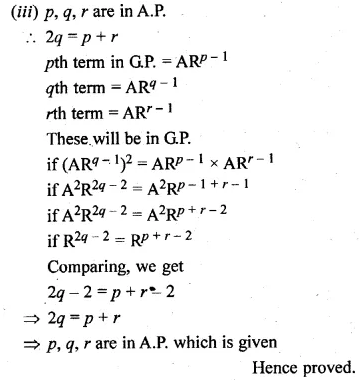Question 28.
If a, b, c are in GP., prove that the following are also in G.P.
(i) a3, b3, c3
(ii) a2 + b2, ab + bc, b2 + c2.
Solution:
(i) a, b, c are in G.P.
∴ b2 = ac
a3, b3, c3 are in G.P.Question 29.
If a, b, c, d are in G.P., show that
(i) a2 + b2, b2 + c2, c2 + d2 are in G.P.
(ii) (b – c)2 + (c – a)2 + (d – b)2 = (a – d)2.
Solution:
a, b, c, d are in G.P.
Let r be the common ratio, then a = a
b = ar, c = ar2, d = ar3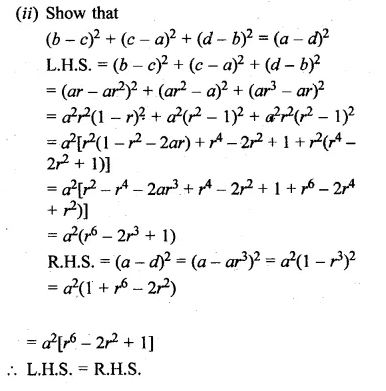Question 30.
The number of bacteria in a certain culture doubles every hour. If there were 30 bacteria present in the culture originally, how many bacteria will be present at the end of 2nd hour, 4th hour and nth hour?
Solution:
Bacteria in the beginning = 30 = a
After every hours, it doubles itself
After 1 hour it becomes = 30 x 2 = 60 = ar
After 2 hours it will becomes = 60 x 2 = 120 = a2
After 3 hours, it will becomes = 120 x 2 = 240 = a3
After 4 hours it will becomes = 240 x 2 = 480 = a4
∴ After n hour, it will become = arn

Question 31.
The length of the sides of a triangle form a G.P. If the perimeter of the triangle is 37 cm and the shortest side is of length 9 cm, find the lengths of the other two sides.
Solution:
Lengths of a triangle are in GP. and its sum is 37 cm
Let sides be a, ar, ar2
a + ar + ar2 = 37
a = 9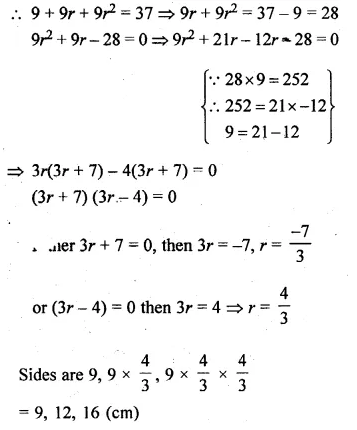Hope given ML Aggarwal Class 10 Solutions for ICSE Maths Chapter 9 Arithmetic and Geometric Progressions Ex 9.4 are helpful to complete your math homework.

If you have any doubts, please comment below. Learn Insta try to provide online math tutoring for you.